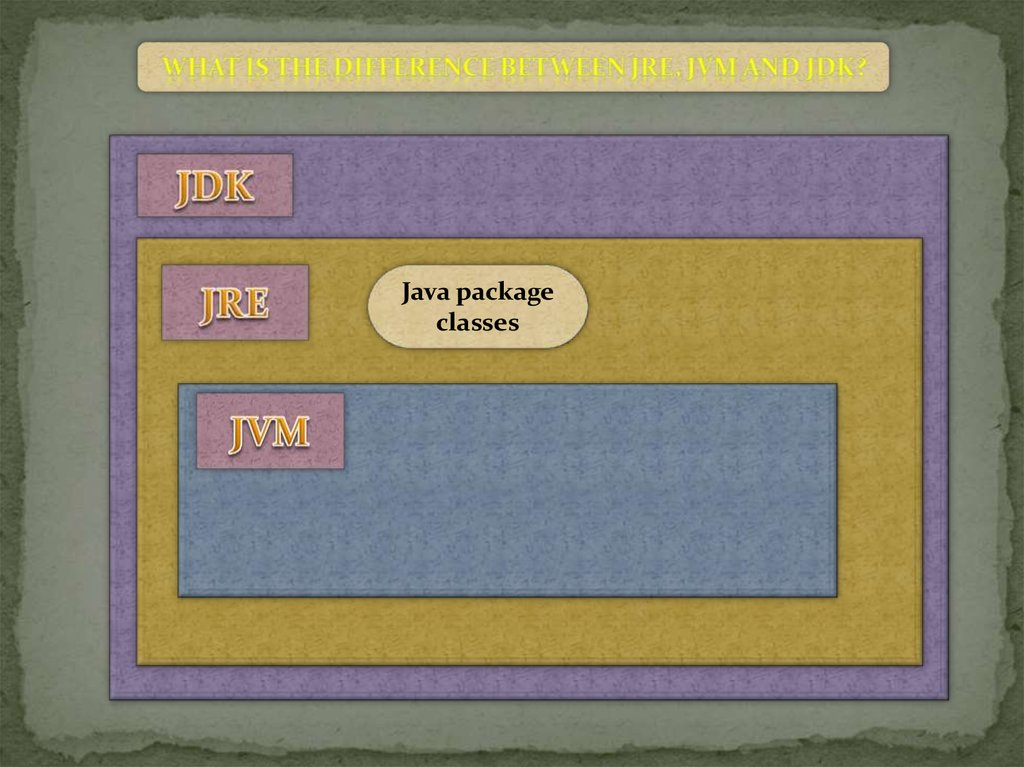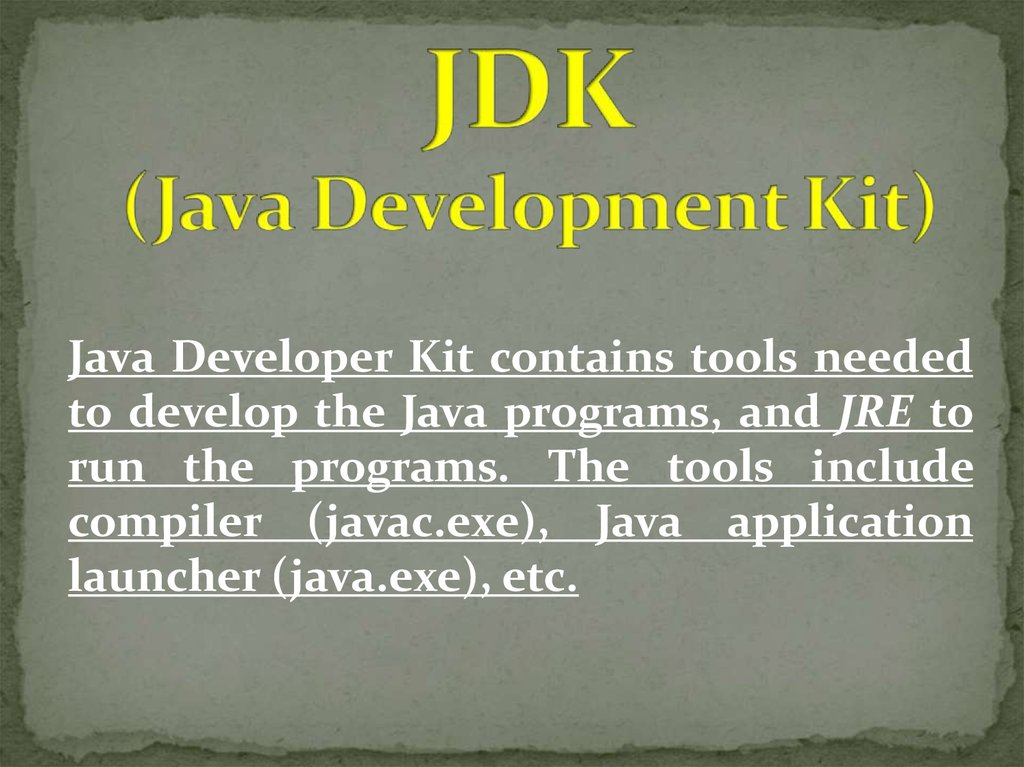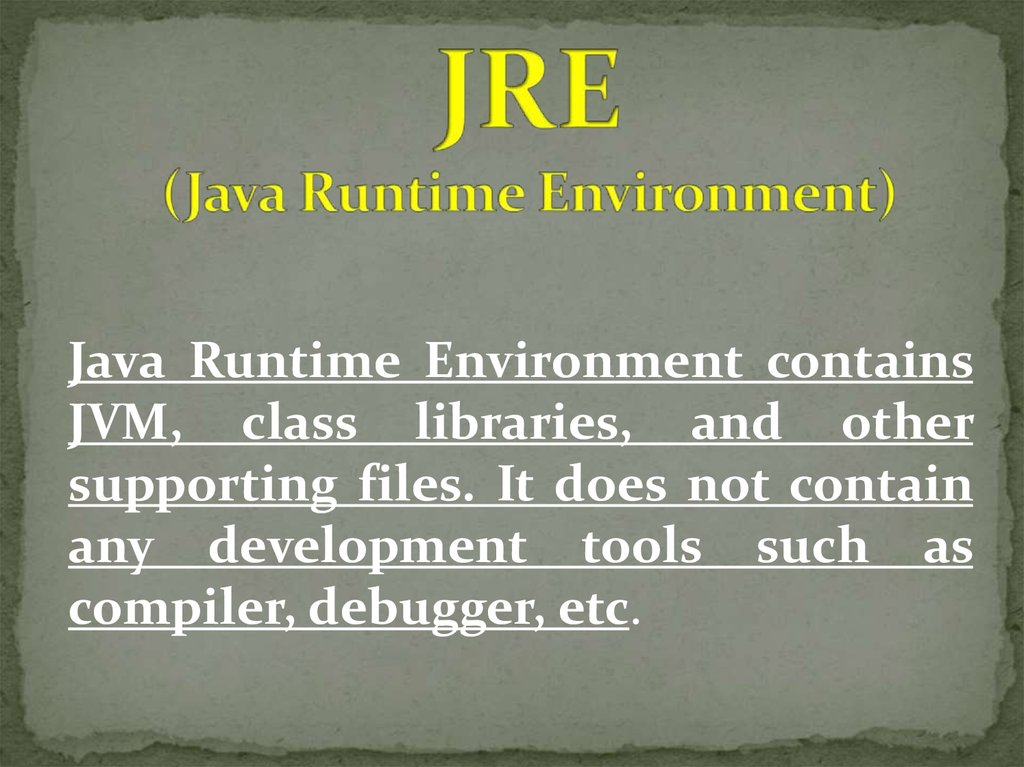# Java package classes

## 1.

Java package
classes
Java package classes

## 2.

Java Developer Kit contains tools needed
to develop the Java programs, and JRE to
run the programs. The tools include
compiler (javac.exe), Java application
launcher (java.exe), etc.

## 3.

Java Runtime Environment contains
JVM, class libraries, and other
supporting files. It does not contain
any development tools such as
compiler, debugger, etc.

## 4.

Java Virtual Machine interprets the
byte code into the machine code
depending upon the underlying
operating system and hardware
combination.

Compiler
(javac.exe)
Debugging
tools
Laucher
(java.exe)
Disassembler
(javap.exe)

## 7.

Interprets the byte code into machine code

## 8.

Creating byte code’s file
By compiler
.java file
(Text file)
.class file
(Byte code)

## 9.

Byte code for initialization of variable
.java file -> .class file(bytecode)
int count; // create variable count
Gets compiled to the following byte code:
0: iconst_0 //Push 0 to top of the operand stack
1: istore_1 //Pop value from top of operand stack and
store as local variable 1

## 10.

Primitive
Types
byte(8 bit, default - 0)
short(16 bits, default - 0)
int(32 bits, default - 0)
long(64 bits, default - 0)
char(16 bits, default – ‘\u0000’)
float(32 bits, default - +0)
double(64 bits, default - +0)
boolean(32 bits, into arrays 8 bit, default
- false)
Reference
Types
• Class types
• Array types
• Interface
types
• Default - null

## 11.

Byte data type is an 8-bit signed two's complement
integer.
Minimum value is -128 (-2^7)
Maximum value is 127 (inclusive)(2^7 -1)
Default value is 0
Byte data type is used to save space in large arrays,
mainly in place of integers, since a byte is four times
smaller than an int.
Example: byte a = 100 , byte b = -50

## 12.

Short data type is a 16-bit signed two's complement
integer.
Minimum value is -32,768 (-2^15)
Maximum value is 32,767 (inclusive) (2^15 -1)
Short data type can also be used to save memory as
byte data type. A short is 2 times smaller than an int
Default value is 0.
Example: short s = 10000, short r = -20000

## 13.

Int data type is a 32-bit signed two's complement
integer.
Minimum value is - 2,147,483,648.(-2^31)
Maximum value is 2,147,483,647(inclusive).(2^31 -1)
Int is generally used as the default data type for
integral values unless there is a concern about
memory.
The default value is 0.
Example: int a = 100000, int b = -200000

## 14.

Long data type is a 64-bit signed two's complement
integer.
Minimum value is -9,223,372,036,854,775,808.(-2^63)
Maximum value is 9,223,372,036,854,775,807
(inclusive). (2^63 -1)
This type is used when a wider range than int is
needed.
Default value is 0L.
Example: long a = 100000L, long b = -200000L

## 15.

Float data type is a single-precision 32-bit IEEE 754
floating point.
Minimum value is 3, 4 * e-038
Maximum value is 3, 4 * e038
Float is mainly used to save memory in large arrays of
floating point numbers.
Default value is 0.0f.
Float data type is never used for precise values such as
currency.
Example: float f1 = 234.5f

## 16.

double data type is a double-precision 64-bit IEEE 754
floating point.
Minimum value is 1,7 * e-308
Maximum value is 1,7 * e308
This data type is generally used as the default data type
for decimal values, generally the default choice.
Double data type should never be used for precise
values such as currency.
Default value is 0.0d.
Example: double d1 = 123.4

## 17.

boolean data type represents one bit of information.
There are only two possible values: true and false.
This data type is used for simple flags that track
true/false conditions.
Default value is false.
Example: boolean one = true

## 18.

char data type is a single 16-bit Unicode character.
Minimum value is '\u0000' (or 0).
Maximum value is '\uffff' (or 65,535 inclusive).
Char data type is used to store any character.
Example: char letterA ='A'